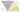## 胡说Eisenstein整环之方程解计数

Fri, 10th January 2020Edit on GithubEisenstein

2010年4月wayne提问 :

# 公式推导

a、b互质时，N有且只能有1(mod3)的质因数。这是数论中关于$Z(\omega)$的一个基本定理。

1、通解公式：$a=|u^2-v^2|, b=2uv, c=u^2+v^2$, 式中GCD(u, v)=1, u, v一奇一偶。
2、计数公式：c只含有1(mod4)的质约数，当c有k个1(mod4)的质约数时，本原(a,b)对有$2^{k-1}$个。
wayne的问题中也有对应的通解公式和计数公式，它们对优化算法的作用应该是完全一样的。

wayne选择特殊的N对hujunhua的结论进行验证:

比如当N=7\times 13\times 19\times 31\times 37\times 43

{a,b}:

{5, 9237}, 		{157, 9312},		 {368, 9413},		{873, 9640},
{880, 9643},		{960, 9677},		{1063, 9720},		{1383, 9848},
{1565, 9917},		{1592,   9927},	{1752, 9985},		{1933, 10048},
{2277, 10160},	{2435,   10208},	{2640, 10267},	{2687, 10280},
{2787, 10307},	{3127, 10392},	{3272, 10425},	{3355, 10443},
{3625, 10497},	{3707,   10512},	{3953, 10553},	{4103, 10575},
{4177, 10585},	{4272, 10597},	{4297, 10600},	{4755, 10643},
{4833, 10648},	{4925, 10653},	{4968, 10655},	{5295, 10663}

mathe认为可以有通解:
i)$(u,v)=1$,
$\begin{cases}b=4uv\\a=3u^2-v^2+2uv\\N=3u^2+v^2\end{cases}$
ii)$(u,v)=1$,
$\begin{cases}b=4uv\\a=u^2-3v^2+2uv\\N=u^2+3v^2\end{cases}$

$\{a, b\}=\{u^2-v^2, 2uv-v^2\}$
$N=u^2-uv+v^2$
$u\gt v\gt 0, Gcd(u,v)=1, u\ne -v(\mod3)$

# Eisenstein整环简介

hujunhua给出: $Z(\omega)$及丢番图方程$a^2-ab+b^2= N^2$简介

## 一、Eisenstein整数环$Z(\omega)$简介。

【定义1】（Eisenstein整数环）$Z(\omega):=\{a+b\omega|a, b\in Z\}$$\omega$是3次单位根，满足$\omega^3=1，\omega^2+\omega+1=0$.

【定义2】（范数）对于$z= a+b\omega，N(z):=|z|^2=(a+b\omega)(a+b\omega^{\prime})=a^2-ab+b^=:a\otimes b$ ，称为$z$的范数。

【定义3】（单位元）范数等于1的Eisenstein整数称为$Z(\omega)$的(乘法)单位元。

【基本性质1】作为环，$Z(\omega)$对加、减、乘法封闭。值得一提的是对乘法封闭的意义，即对任意的a+b\omega与c+d\omega, 存在e+f\omega=(a+b\omega)(c+d\omega)。由于降次公式\omega[sup]2[/sup]=-1-\omega，这是显然的。取范数可得代数恒等式 $(a\otimes b)(c\otimes d)=(ac-bd)\otimes (da+bc+bd)$ 胡子评曰：恒等式“两平方和乘积=两平方和”与此具有相似的背景和意义，它们在初等代数中都不是显然的，仿佛灵光一闪间妙手偶得之，但在Z(i)和$Z(\omega)$中一点也不惊奇，就跟1+1=2差不多。从高端看初等的优势于此可见一斑。 【基本性质2】$Z(\omega)$中成立算术基本定理，并且是唯一分解的整环。

2) $a+b\omega, a\otimes b=1(mod6)$的素数。即6m+1形的自然素数都不是$Z(\omega)$中的素数，都可以唯一地分解为$Z(\omega)$中一对共扼素数$a+b\omega$$a+b\omega^{\prime}$的积。
3) 2(mod3)的自然素数， 即2和6m-1形自然素数在$Z(\omega)$仍然是素数。

## 二、几个对本帖有用的引理。

【引理1】 $\omega\equiv\omega^{\prime}\equiv1(\mod\rho)$

【引理2】 $Gcd(a+b\omega, a+b\omega^{\prime})|\rho Gcd(a, b)$

$=Gcd(\rho a, \rho b)=\rho Gcd(a, b)$.

【引理3】 $d|(a+b\omega)$, 当且仅当$d|Gcd(a,b)$. 证：d总是同时整除$a+b\omega$$a+b\omega^{\prime}$，即$d|Gcd(a+b\omega, a+b\omega^{\prime})|\rho Gcd(a, b)$. 当$d$不含因子3时，显然$d|Gcd(a,b$). 当$3|d$时，因$d$$Gcd(a, b)$均含有偶数个$\rho$，仍有$d|Gcd(a,b)$.

## 三、丢番图方程$a^2+ab+b^2= n^2$的通解公式和解数

    除了那些基本性质，关于$Z(\omega)$最容易想到的一个问题就是哪些$a+b\omega(ab\ne 0)$的模为自然整数，即范数$N(a+b\omega)$为自然平方数。这就导致方程:  

$a\otimes b=n^2$. 在$Z(\omega)$中写为$(a+b\omega)(a+b\omega^{\prime})=n^2$.

1）n不含任何第3)类素数因子$p^3$. 否则$p^3|(a+b\omega)$，由引理3得$p^3|Gcd(a, b)=1$，矛盾.
2）n不含因子3. 否则$n^2$$\rho^4$. 由于引理1的推论1知$a+b\omega$$a+b\omega^{\prime}$平分$n^2$中的$\rho$，那么至少各分得$\rho^2$，即$3|Gcd(a, b)=1$，矛盾. 于是按引理2推论2可得
3) $Gcd(a+b\omega, a+b\omega^{\prime})=Gcd(a,b)=1$，因此总可得到$a+b\omega=(u+v\omega)^2$$a+b\omega^{\prime}=(u+v\omega^{\prime})^2$$n=u\otimes v$.

4)假定$n=p_1^{s_1}\cdot p_2^{s_2}\cdot \cdot \cdot p_i^{s_i}\cdot \cdot \cdot p_k^{s_k}$$p_i=(a_i+b_i\omega )(a_i+b_i\omega^{\prime})$，为了构造$a+b\omega$$a+b\omega^{\prime}$，我们需要将$(a_i+b_i\omega)^{2s_i}(a_i+b_i\omega^{\prime})^{2s_i}$的所有质因子（按重数计）平分成两堆，划拨给$a+b\omega$$a+b\omega^{\prime}$。由于引理3的原因，$a+b\omega$不能同时要一对共扼因子，$a+b\omega^{\prime}$也一样。否则短路打火，烧焦成实因子，就破坏了a与b的互质性。所以要么将$(a_i+b_i\omega )^{2s_i}$全部给$a+b\omega$$(a_i+b_i\omega^{\prime})^{2s_i}$全部给$a+b\omega^{\prime}$，要么将$(a_i+b_i\omega^{\prime})^{2s_i}$全部给$a+b\omega$$(a_i+b_i\omega)^{2s_i}$全部给$a+b\omega^{\prime}$，只有这2种分配方案。于是全部分配方案就是$\frac{2^k}2=2^{k-1}$种。除以2是因为共扼对称和乘法交换律。

Github